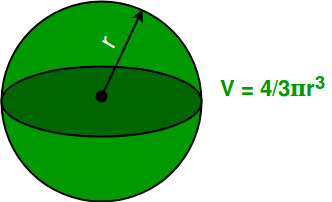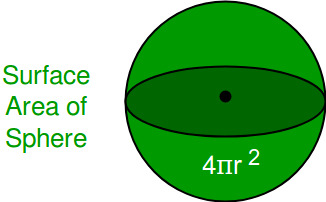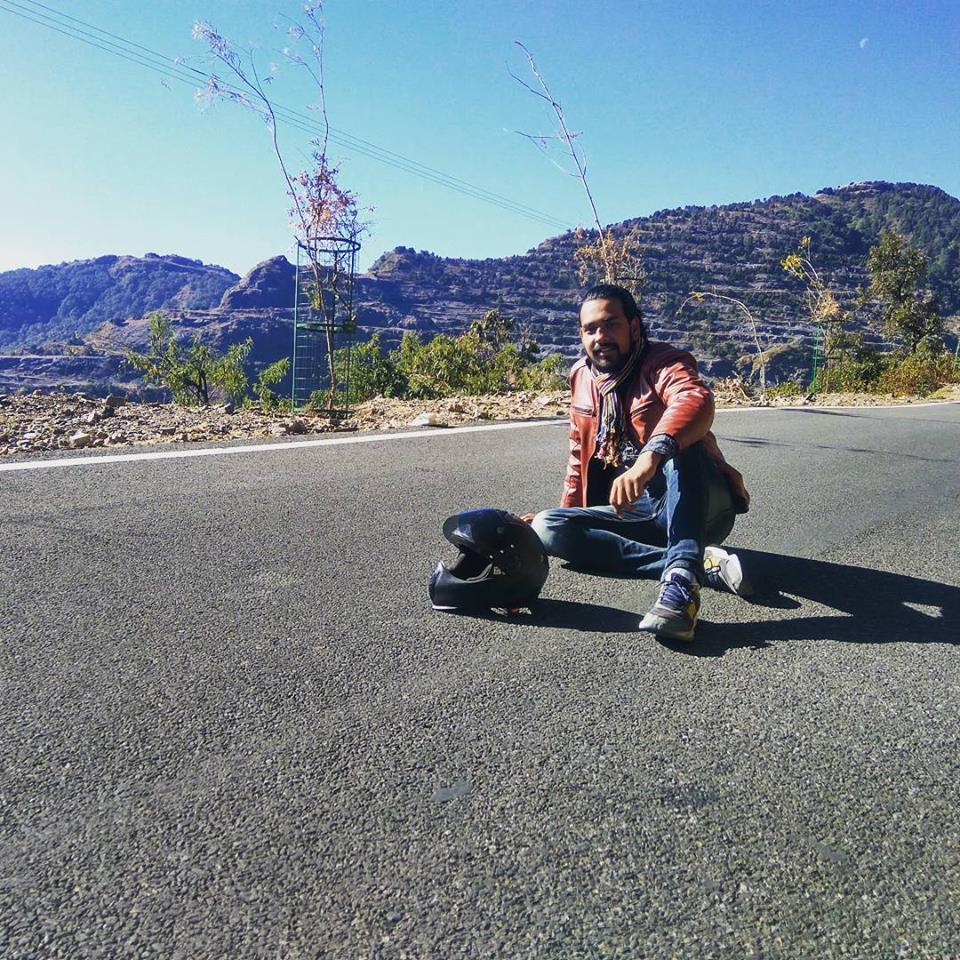# Calculate Volume and Surface area Of Sphere

Given radius of sphere, calculate the volume and surface area of sphere.

Sphere:
Just like a circle, which geometrically is a two-dimensional object, a sphere is defined mathematically as the set of points that are all at the same distance r from a given point, but in three-dimensional space. This distance r is the radius of the sphere, and the given point is the center of the sphere.
For a given surface area, the sphere is the one solid that has the greatest volume. This why it appears in nature so much, such as water drops, bubbles and planets etc.

Volume Of Sphere:
The number of cubic units that will exactly fill a sphere or the storage capacity of sphere. We can calculate the volume of sphere by using formula:Surface Area Of Sphere:
The surface area of a sphere object is a measure of the total area that the surface of the sphere occupies. We can calculate the volume of sphere by using formula:Examples :

```Input  : Radius Of Sphere = 5
Output : Volume    Of    Sphere :  523.5987755982989
Surface Area Of Sphere :  314.1592653589793
Explanation:
Volume =( 4/3 ) * 3.14159 * 5 * 5 * 5 = 523.598
Surface Area = 4 * 3.14159 * 5 * 5 =314.159

Input  : Radius Of Sphere = 12
Output : Volume    Of    Sphere :  7238.229473870883
Surface Area Of Sphere :  1809.5573684677208

```

## C++

 `// CPP program to calculate Volume and  ` `// Surface area of Sphere ` `#include ` `using` `namespace` `std; ` ` `  `// Initializing Value Of PI ` `float` `pi = 3.14159; ` ` `  `// Function To Calculate Volume Of Sphere ` `float` `volume(``float` `r) ` `{ ` `    ``float` `vol; ` `    ``vol = (``float``(4) / ``float``(3)) * pi * r * r * r; ` `    ``return` `vol; ` ` `  `} ` ` `  `// Function To Calculate Surface Area of Sphere ` `float` `surface_area(``float` `r) ` `{ ` `    ``float` `sur_ar; ` `    ``sur_ar = 4 * pi * r * r; ` `    ``return` `sur_ar; ` `} ` ` `  `// Driver Function ` `int` `main() ` `{ ` `    ``float` `radius = 12; ` `    ``float` `vol, sur_area; ` `     `  `    ``// Function Call ` `    ``vol = volume(radius); ` `    ``sur_area = surface_area(radius); ` ` `  `    ``// Printing Value Of Volume And Surface Area ` `    ``cout << ``"Volume Of Sphere :"` `<< vol << endl; ` `    ``cout << ``"Surface Area Of Sphere :"` `<< sur_area << endl; ` `    ``return` `0; ` `} `

## Java

 `// Java program to calculate Volume and ` `// Surface area of Sphere ` `class` `GFG { ` `     `  `// Initializing Value Of PI ` `static` `float` `pi = ``3``.14159f; ` ` `  `// Function To Calculate Volume Of Sphere ` `static` `float` `volume(``float` `r) ` `{ ` `    ``float` `vol; ` `    ``vol = ((``float``)``4` `/ (``float``)``3``) * (pi * r * r * r); ` `    ``return` `vol; ` `} ` ` `  `// Function To Calculate Surface Area of Sphere ` `static` `float` `surface_area(``float` `r) { ` `    ``float` `sur_ar; ` `    ``sur_ar = ``4` `* pi * r * r; ` `    ``return` `sur_ar; ` `} ` ` `  `// Driver Function ` `public` `static` `void` `main(String[] args)  ` `{ ` `    ``float` `radius = ``12``; ` `    ``float` `vol, sur_area; ` ` `  `    ``// Function Call ` `    ``vol = volume(radius); ` `    ``sur_area = surface_area(radius); ` `     `  `    ``// Printing Value Of Volume And Surface Area ` `    ``System.out.println(``"Volume Of Sphere :"` `+ vol); ` `    ``System.out.println(``"Surface Area Of Sphere :"` `+ sur_area); ` `} ` `} ` ` `  `// This code is contributed by Anant Agarwal. `

## Python3

 `''' Python3 program to calculate Volume and  ` `Surface area of Sphere'''` `# Importing Math library for value Of PI ` `import` `math ` `pi ``=` `math.pi ` ` `  `# Function to calculate Volume of Sphere ` `def` `volume(r): ` `    ``vol ``=` `(``4` `/` `3``) ``*` `pi ``*` `r ``*` `r ``*` `r ` `    ``return` `vol ` ` `  `# Function To Calculate Surface Area of Sphere ` `def` `surfacearea(r): ` `    ``sur_ar ``=` `4` `*` `pi ``*` `r ``*` `r ` `    ``return` `sur_ar ` ` `  `# Driver Code ` `radius ``=` `float``(``12``) ` `print``( ``"Volume Of Sphere : "``, volume(radius) ) ` `print``( ``"Surface Area Of Sphere : "``, surfacearea(radius) ) `

## C#

 `// C# program to calculate Volume and ` `// Surface area of Sphere ` `using` `System; ` ` `  `class` `GFG { ` `     `  `    ``// Initializing Value Of PI ` `    ``static` `float` `pi = 3.14159f; ` `     `  `    ``// Function To Calculate Volume ` `    ``// Of Sphere ` `    ``static` `float` `volume(``float` `r) ` `    ``{ ` `        ``float` `vol; ` `        ``vol = ((``float``)4 / (``float``)3) *  ` `                           ``(pi * r * r * r); ` `        ``return` `vol; ` `    ``} ` `     `  `    ``// Function To Calculate Surface Area  ` `    ``// of Sphere ` `    ``static` `float` `surface_area(``float` `r) { ` `        ``float` `sur_ar; ` `        ``sur_ar = 4 * pi * r * r; ` `        ``return` `sur_ar; ` `    ``} ` `     `  `    ``// Driver Function ` `    ``public` `static` `void` `Main()  ` `    ``{ ` `        ``float` `radius = 12; ` `        ``float` `vol, sur_area; ` `     `  `        ``// Function Call ` `        ``vol = volume(radius); ` `        ``sur_area = surface_area(radius); ` `         `  `        ``// Printing Value Of Volume And  ` `        ``// Surface Area ` `        ``Console.WriteLine(``"Volume Of Sphere :"` `                                        ``+ vol); ` `        ``Console.WriteLine(``"Surface Area Of "`  `                      ``+ ``"Sphere :"` `+ sur_area); ` `    ``} ` `} ` ` `  `// This code is contributed by vt_m. `

## PHP

 ` `

Output :

```
Volume Of Sphere :7238.22
Surface Area Of Sphere :1809.56

```

My Personal Notes arrow_drop_upCheck out this Author's contributed articles.

If you like GeeksforGeeks and would like to contribute, you can also write an article using contribute.geeksforgeeks.org or mail your article to contribute@geeksforgeeks.org. See your article appearing on the GeeksforGeeks main page and help other Geeks.

Please Improve this article if you find anything incorrect by clicking on the "Improve Article" button below.

Improved By : vt_m